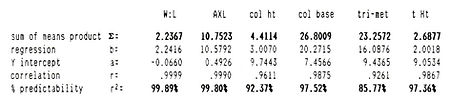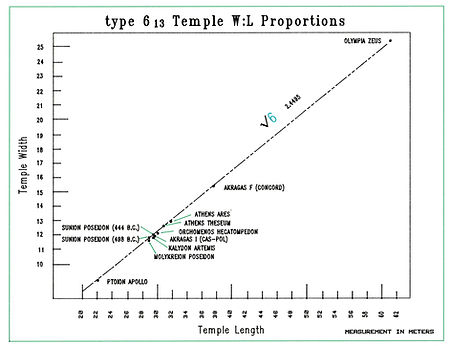top of page#### Results and AnalysisThe statistical results show the strongest linear relationship exists between temple interaxial width and length. More specifically, with every increase in temple width, there is an exact and corresponding increase in the length. It also shows the independent variables (temple width) have a remarkable predictive capacity (r), for the dependent variable temple length.

The breakdown of the type temples under investigation indicate that type 6 12 temples have a noticeable break in the ratio continuity after Aegina Aphaia ca. 490 B.C., the usually accepted terminus between the Archaic and Classical periods. Those type 612 Archaic temples of Metapontum Hera (b=2.1769), Delphi Athena (b=2.1704), and Hermione Poseidon (b=2.1795) all have width/length ratios that are best expressed in a ratio of @13:6 or 2.175.  Neither Tobin’s [1981:348] Archaic rule nor J.J. Coulton’s [1974:83] “Sicilian rule” adequately explain these devient examples which clearly break from the rest of the temple group with a ratio of 2.2367. A similar phenomenon occures in type 613 temples where a discrete break occures between the Archaic and Classical periods (535 B.C. and 490 BC), when all temples begin to conform to a new and rigidly structured proportional rule.

The exmple of Athena at Assos (560 B.C.) for one, has a width/length ratio that is 8% deviated from the norm. The remaining Archaic temples within this class (Isthmia Poseidon 460 BC, W:L = 2.4054; Delos Apollo 475 BC, W:L = 2.3999; and Paestum Athena 530 BC, W:L = 2.3972), appear to follow the Tobin rule of Archaic temple proportions (12:5 = 2.40), all other type 6 13 temples follow a proportional mean that adhears to a value of 2.4456. At the surface, then, one could make the assumption that E.F Winter [1978:153] makes:  “…at least during the Archaic period, there was no consistent and orderly development of temple plans….”

If one agrees with Winter, Archaic period buildings could be removed from the data set and the numbers recalculated.The results of the Classical Period temple statistical correlations show an exceptionally high confidence of predictability for each Type 6 sub-type group. These results, however, contradict the view that Classical architects did not use “…specific rules of proportion [or] have a theoretical basis [in their design,” [Coulton, 1975:67-68].

What can be determined from the results is that temple peripteral dimensions, as defined by the axial peristyle at least, seemed to have formed the initial step in temple construction.  Colton’s [1974:66] idea “…that once the stylobate dimensions had been defined, they were regarded as fixed dimensions to which the rest of the design must be adjusted” seems to be accurate.   Although even with the aesthetic modifications of end column contractions, there appears to be no variation in the proportional rule for Classical period temple design.  This applies to all Classical period type temples throughout the Mediterranean with no distinction between geographic regions.

The significance of the temple width x length proportions encourage us to look at the other measurements and dimensions. When examining column heights for example, it is clear there is a recognizable pattern. The graph below shows that for every unit increase in ‘x’ there is a 1.5m unit increase in ‘y’. Subsequently, the predictability of the column can be assigned a reasonable value based on the statistical outcome. A case in point is the Hecatompedon at Orchomenos. Using the formula of 1.5 units of height for each unit of temple length, a column height of 6.0 meters is arrived at given a interaxial length of 30.20 meters. Likewise, a column height for the temple of Poseidon at Isthmia would be about 8.9 meters.

It should also be noted that temples segragated because of thier Archaic time period, have column heights that fall far short in stature from their Classical period cousins. The shorter, squater columns confirm that there was not a recognized building standard from which they could draw upon. The temple of Assos Athena (470 BC) has a column height of 4.58 meters, significantly shorter (1.02 meters shorter) than the statistical mean. Likewise, temple D at Selinunte has columns 1.14 meters shorter than the statistical mean.With regard to column heights for Archaic period temples, J-P Adams (Temple de Hera, a Paestum, Rev. Arch. 2[1973:219-236]) had a diagram of the temple of Hera II (Poseidon) at Paestum. A portion of that diagram employed an interesting geometric calculations showing a connection between column spacing to that of the column heights. In order to test his calculation, I used temple D at Selinunte, especially since that temple showed a significantly short stature to its columns. According to Adams’ diagram, the column height might have been determined by drawing an arc between three columns; column one being the interaxial starting point and column two separating the others, with the third column being the terminus of the arc at the interaxial height of the column abacus. The significance of the diagram is that the formula seems to work.

In the case of temple D at Selinunte, the column height is measured at 8.94 meters. If we recreate the diagram used by Adams, we see that the column height comes out exactly as it is predicted. This seems to be a useful tool for determining column heights for archaic temples, although more research is needed to make it the rule.bottom of page